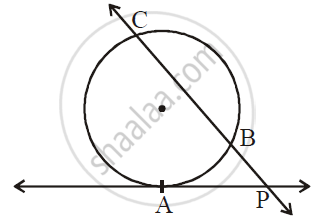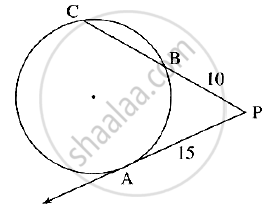SSC (English Medium) Class 10th Board ExamMaharashtra State Board
Share

# In the Following Figure, Ray Pa is a Tangent to the Circle at a and Pbc is a Secant. If Ap = 15, Bp = 10, Then Find Bc. - SSC (English Medium) Class 10th Board Exam - Geometry

#### Question

In the following figure, ray PA is a tangent to the circle at A and PBC is a secant. If AP = 15, BP = 10, then find BC.#### SolutionIn the given figure, PA is a tangent segment and PBC is the secant

∴ PB x PC = PA2

:. 10 xx PC = 15^2

:. PC = 225/10 = 22.5

NOw PB + BC = PC  .............(P - B - C)

∴ 10 + BC = 22.5

∴ BC = 22.5 - 10 = 12.5

Therefore BC = 12.5

Is there an error in this question or solution?

#### Video TutorialsVIEW ALL 

Solution In the Following Figure, Ray Pa is a Tangent to the Circle at a and Pbc is a Secant. If Ap = 15, Bp = 10, Then Find Bc. Concept: Tangent - Secant Theorem.
S